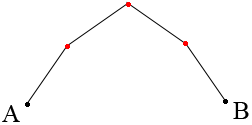SEARCH HOMEMath Central Quandaries & QueriesQuestion from tammie, a student: Koch's kinky curve is created by starting with a straight segment and replacing it with four segments, each 1/3 as long as the original segment. So, at the second stage the curve has three bends. At the next stage, each segment is replaced by four segments, and so on. How many bends does the curve have at the third stage?Hi Tammie,

I drew a diagram of what I think you have at stage 2. The original line segment was from A to B and now there are four segments with bends at the points where I have put a red dot.For stage 3 replace each of the four line segments by smaller versions of the curve from A to B in stage 2. Count the number of bends you have. Can you see how you might have found the number of bends without the diagram? How many bends are there in stage 4?

PennyMath Central is supported by the University of Regina and the Imperial Oil Foundation.